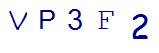# Why 8th Grade Geometry Is Pivotal in Your Child’s Future Math PerformanceLast Updated on May 31, 2022 by Thinkster

Is your child having trouble with 8th grade geometry concepts being introduced during this crucial school year? If so, then it’s time to consider getting some help. These concepts are simply too important to let your child slide by without proper understanding, and here’s why.

### 8th Grade Geometry Concepts Build Important Foundations

The concepts taught in geometry in the 8th grade math class are foundational for future understanding of geometry concepts. If students are not able to grasp these concepts, they will struggle in future math classes. This is the first year, for example, that students will make proofs to prove that something is true. They will also learn to manipulate two- and three-dimensional shapes and angles. These concepts will be built on in later math classes and also in real-world problem-solving in the future.

### Geometry and Algebra Combine in 8th Grade

8th grade geometry concepts are often overlapped by algebra concepts. For instance, the coordinate plane is an important part of algebra class, but also has some applications in geometry. This is why it is so vital for eighth graders to get a solid foundation in their geometry classes.

### 8th Grade Geometry Introduces Valuable Vocabulary

In 8th grade, students will have their first experiences with important geometry vocabulary. Some of these terms include:

• Rotation
• Reflection
• Translation
• Line
• Line Segment
• Point
• Plane
• Dilation

A basic understanding of these terms is required to succeed in high school geometry class, so it’s important that the right foundation is laid in middle school.

### 8th Grade Geometry Introduces Important Formulas

How many times in high school math class did you work with the Pythagorean Theorem? This important concept is now introduced in 8th grade according to the Common Core Standards. If your child is struggling to comprehend this important formula, future math instruction will also be a struggle.

Yet this is just one important formula introduced in 8th grade geometry. Students will also learn formulas for the volume of cones, spheres, and cylinders. This is a math concept that has real-world applications that your child will need in adult life, as well as in future math classes. Eighth graders will also gain additional practice in the formulas used for perimeter and area of all basic shapes.

As you can see, 8th grade geometry is incredibly important. If your child is struggling, get help before the struggle becomes too serious. Thinkster is here to support the mathematical understanding of students through 8th grade using an online math tutoring program.

SummaryArticle Name
Why 8th Grade Geometry Is Pivotal in Your Child's Future Math Performance
Description
Find out why 8th grade geometry is crucial to your child's future and how you can help them excel at it.
Author
Publisher Name
Thinkster Math
Publisher Logo

## Subscribe to Thinkster Blog## Recommended Articles### Unlocking the Code: Teaching Mathematical Symbols and Their Meaning

Knowledge of mathematical symbols is a foundational skill that empowers students to engage with and excel i...